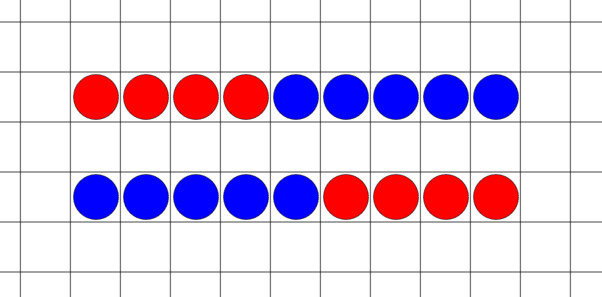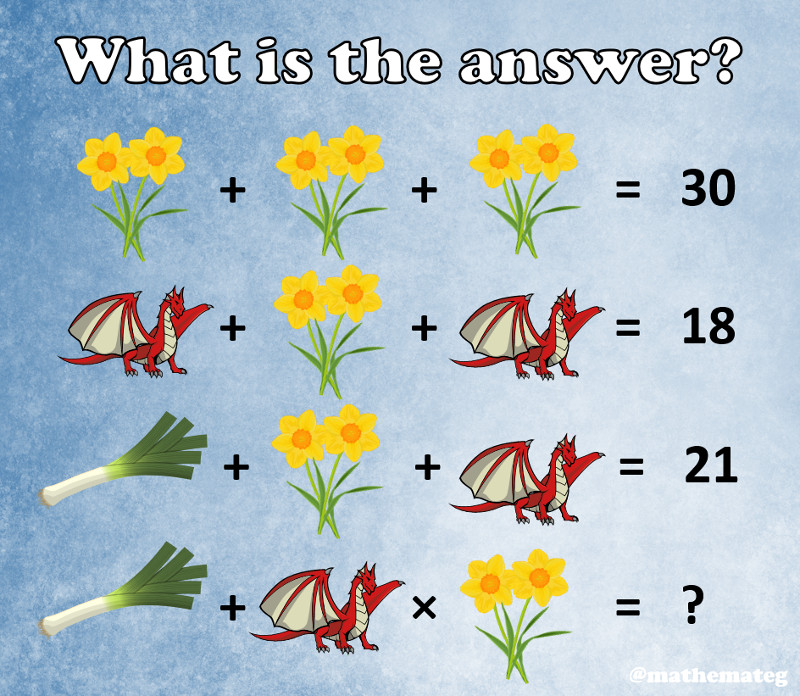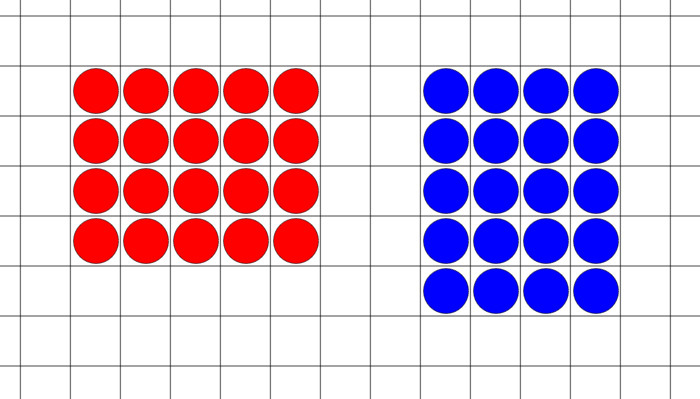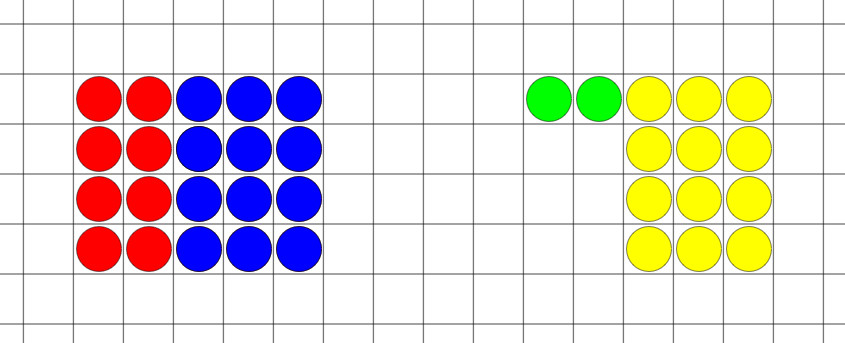## Commutativity and the order of operationsWelsh version of the blog

The word “commutativity” appears in several places in the new draft Mathematics and Numeracy AOLE, for example as part of the second What Matters statement.

Progression Step 2

“I have explored commutativity with addition and multiplication and I can recognise when two different numerical expressions describe the same situation but are written in different ways.”

Progression Step 3

“I can recognise equalities, inequalities and equivalence of expressions, and also when commutativity, distributivity and associativity can be used to state a simple expression in a different way.”

Technical vocabulary such as the word “commutativity” can sometimes lead to concerns regarding the accessibility of the curriculum document, so this blog will explore the idea of commutativity and motivate its inclusion in the curriculum.

What is commutativity?

Commutativity relates to the commutative law, a law used in mathematics to describe operators that give the same answer, even if the order in which we input the numbers is changed. For example, the addition of numbers is commutative, as can be seen in the example 4 + 5 = 5 + 4.One goal of the new curriculum is to encourage teachers to teach for understanding, and not to rely on tricks and shortcuts. One commonly used trick is the acronym BODMAS, an acronym used to help remember the order in which operations should be performed. (Other acronyms, such as BIDMAS or PEMDAS, are also in use.) The misapplication (or sometimes plain unawareness of!) BODMAS is often seen in the comments to social media posts such as the one shown below.Here, having worked out that a pair of daffodils is 10, a dragon is 4, and a leek is 7, we are faced with having to calculate

7 + 4 × 10

A common mistake is to work left-to-right, calculating 7 + 4 first and then calculating 11 × 10 to obtain the answer 110. The correct answer requires us to perform the multiplication first (4 × 10 = 40), and then calculate 7 + 40 to obtain 47. How is this (the order of operations) taught in schools? I would suspect (and I have been guilty of this in the past) a common approach of presenting the order of operations, perhaps using an acronym such as BODMAS, and then asking students to complete a set of exercises, for example

a)       2 + 3 × 4

b)      9 ÷ 3 + 5

c)       9 – 3 + 4

Pupils can generally answer correctly a set of exercises such as the one above, given that some teacher instruction has occurred before hand. Using the proficiencies of the new curriculum, we could say that by completing the above exercise successfully, pupils have started to show some fluency in using the correct order of operations. A quite different consideration would be to ask whether pupils have good conceptual understanding of the order of operations? For this, we would have to ask whether pupils understand why operations have to be performed in a certain order in the first place. For example, why does multiplication need to be performed before addition? It turns out that commutativity helps us understand this.

Let us consider the previous addition example,

4 + 5 = 5 + 4

We can agree that both sides of this equation have the same answer, namely 9. By replacing the 4 on both sides by 2 × 2, we arrive at

2 × 2 + 5 = 5 + 2 × 2

For the left-hand side, working left-to-right, we need to calculate 2 × 2 first, to obtain 4, and then calculate 4 + 5 to reach 9. This agrees with the answer from before. For the right-hand side however, we see that working left-to-right will not work, as this leads us to add 5 and 2 to begin with, to obtain 7, and then calculate 7 × 2 to obtain 14. This is obviously different to what we intended (9), so we are motivated to state that multiplication should take precedence over addition. This way, the commutative law for addition cannot be broken.

5 + 2 × 2
= 5 + 4
= 9

Let us now consider another example, the multiplication sum 4 × 5. As the multiplication of numbers is commutative, we can state that

4 × 5 = 5 × 4We can agree that both sides of this equation have the same answer, namely 20. By replacing the 5 on both sides by 3 + 2, we arrive at

4 × 3 + 2 = 3 + 2 × 4

Having decided previously that multiplication should take precedence over addition, the left-hand side comes to 12 + 2 = 14, whereas the right-hand side comes to 3 + 8 = 11. None of these are equal to 20! Having addition take precedence over multiplication would fix things, but this would contradict our earlier decision to have multiplication take precedence over addition. The solution is to introduce a pair of brackets (or parentheses), and write

4 × (3 + 2) = (3 + 2) × 4

Both sides now give 20, as long as we insist that any sum in brackets takes precedence over both multiplication and addition. This way, the commutative law for multiplication cannot be broken.

Summary so far:

• To preserve the commutative law for addition, multiplication must take precedence over addition.
• To preserve the commutative law for multiplication, introduce brackets and perform any calculations in brackets first.

At this point it would be appropriate to comment that the order of operations is just a convention – it is a set of choices that have been made and agreed upon. Had it been chosen that addition takes preference over multiplication, then our multiplication example

4 × 3 + 2 = 3 + 2 × 4

would work just fine without brackets, but our addition example

2 × 2 + 5 = 5 + 2 × 2

would need brackets to become correct:

(2 × 2) + 5 = 5 + (2 × 2)

As it is, multiplication has been chosen to take preference over addition, due to the reason that we are required to write less brackets using this convention. For example, imagine writing the polynomial 6x² + 5x + 4 if addition took preference over multiplication; it would need to be written as (6x²) + (5x) + 4.

The curse of BODMAS

A ‘trick’ such as the acronym BODMAS can lead to success in the short term, but cause misconceptions and problems in the long term. The biggest problem is that students will often just forget about it, and work left-to-right when faced with a sum such as 2 + 3 × 4. A better conceptual understanding of the order of operations will lessen the likelihood of this happening. This may be in the form of the student recognising that 2 + 3 × 4 must have the same answer as 3 × 4 + 2 (using the commutative law for addition). It may be in the form of being able to draw a picture of the situation, realising that (2 + 3) × 4 is different to 2 + (3 × 4).It may also be in the form of more exposure to this type of problem, be it more time spent discussing it the first time in class, or by careful interleaving of the topic in subsequent lessons (spaced over days, months and eventually years).

Another problem with BODMAS is that some students think that the letters in the acronym are strictly ordered, so that (for example) addition must always be performed before subtraction. Thus, when faced with the sum

9 – 3 + 4

students ‘strictly’ following BODMAS will perform 3 + 4 first, to obtain 7, and then do 9 – 7 to obtain 2. In reality, addition and subtraction do not take precedence over each other, and must be performed left-to-right. This way, the correct way to calculate the sum 9 – 3 + 4 is

9 – 3 + 4
= 6 + 4
= 10

In conclusion, certain terms (such as ‘commutativity’) have been placed in the curriculum for a reason. They are not there to make the curriculum intentionally full of jargon, but to promote discussion and drive better classroom practice. The challenge undoubtedly now is to ensure sufficient professional development so that each teacher can be made aware of, and take full advantage of, the opportunities presented by the new curriculum.

Ideas to try in the classroom:

• Try to type 3 + 4 × 5 on the Windows PC calculator program. Why are the answers in standard mode and scientific mode different?
• Is it possible to eliminate the need for a convention of order of operations if enough brackets are included in a calculation?
• Try to design a puzzle (similar to the daffodils, dragon, leek example in this blog) to publish on the school’s social media channels.

Diweddarwyd ddiwethaf: Mercher, 10 Gorffennaf 2019, 8:19 pm